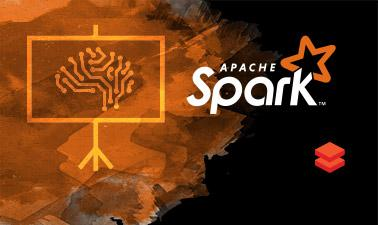# Distributed Machine Learning with Apache Spark

Learn the underlying principles required to develop scalable machine learning pipelines and gain hands-on experience using Apache Spark.This course is archived
Estimated 4 weeks
5–10 hours per week
Instructor-paced
Instructor-led on a course schedule

Machine learning aims to extract knowledge from data, relying on fundamental concepts in computer science, statistics, probability and optimization. Learning algorithms enable a wide range of applications, from everyday tasks such as product recommendations and spam filtering to bleeding edge applications like self-driving cars and personalized medicine. In the age of ‘big data’, with datasets rapidly growing in size and complexity and cloud computing becoming more pervasive, machine learning techniques are fast becoming a core component of large-scale data processing pipelines.

This statistics and data analysis course introduces the underlying statistical and algorithmic principles required to develop scalable real-world machine learning pipelines. We present an integrated view of data processing by highlighting the various components of these pipelines, including exploratory data analysis, feature extraction, supervised learning, and model evaluation. You will gain hands-on experience applying these principles using Spark, a cluster computing system well-suited for large-scale machine learning tasks, and its packages spark.ml and spark.mllib. You will implement distributed algorithms for fundamental statistical models (linear regression, logistic regression, principal component analysis) while tackling key problems from domains such as online advertising and cognitive neuroscience.

### At a glance

• Institution: BerkeleyX
• Subject: Computer Science
• Level: Intermediate
• Prerequisites:
• Python programming background
• experience with PySpark equivalent to CS105x: Introduction to Spark
• comfort with mathematical and algorithmic reasoning
• familiarity with basic machine learning concepts
• exposure to algorithms, probability, linear algebra and calculus
• Language: English
• Associated programs:

# What you'll learn

Skip What you'll learn
• The underlying statistical and algorithmic principles required to develop scalable real-world machine learning pipelines
• Exploratory data analysis, feature extraction, supervised learning, and model evaluation
• Application of these principles using Spark
• How to implement distributed algorithms for fundamental statistical models